## 3.2.1 Equilibrium Ensemble Average

At finite temperatures, one assumes that the particle, either electron or phonon, is interacting with a bath of other particles. The exact state of all these other particles is not known, since they are fluctuating between different configurations. At finite temperature under thermodynamic equilibrium the state of a system is described by the equilibrium density operator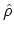(see Appendix C). In treating such systems, it will be most convenient to use the grand canonical ensemble, which allows for a variable number of particles. Therefore, the system is considered to be in contact with a heat bath of temperature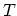and a particle reservoir characterized by the FERMI energy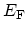. With the definition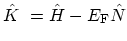, where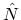is the particle number operator, the statistical operator can be written as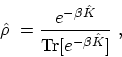(3.10)

where the short-hand notation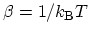is used. The operator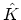may be interpreted as a grand canonical HAMILTONian. Given the density operator, the ensemble average of any operator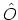can be calculated as(3.11)

Therefore, the single-particle GREEN's function at finite temperature can be defined as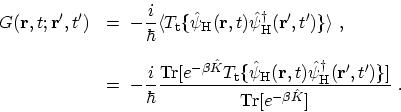(3.12)

At this stage, this form of the GREEN's function does not admit the WICK decomposition, because the WICK theorem described in Section 3.4.1 requires a dependence on the non-interacting HAMILTONian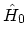for both the field operators and the thermal average. A way around this problem is the MATSUBARA technique , where one introduces a complex timeand a new physical quantity, the MATSUBARA (imaginary time) GREEN's function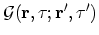. The representation of operators with imaginary time arguments is given in Appendix B.5. M. Pourfath: Numerical Study of Quantum Transport in Carbon Nanotube-Based Transistors